# EM.Ferma Tutorial Lesson 2: Analyzing A Parallel Plate Capacitor

 Tutorial Project: Analyzing A Parallel Plate Capacitor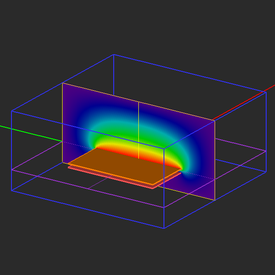Objective: In this project, you will analyze parallel plate capacitor structures made up of metal and dielectric parts. Concepts/Features: CubeCAD PEC Objects Dielectric Objects Computational Domain Fixed-Potential PEC Field Sensor Observable Field Integral Capacitance Minimum Version Required: All versions

## What You Will Learn

In this tutorial you will construct structures involving metal and dielectric objects in EM.Ferma, visualize their electric field and potential and calculate their capacitance. Specifically, you will build and analyze air-filled and dielectric-filled parallel plate capacitors.

## Getting Started

Open the EM.Cube application and switch to EM.Ferma. Start a new project with the following parameters:

 Name EMFerma_Lesson2 Millimeters N/A (keep default) N/A (keep default) N/A (keep default)

## Creating the Metal Plate Objects

in EM.Ferma, perfect electric conductors (PEC) represent equipotential surfaces, bodies or objects. A PEC group has a color and a "Fixed Potential" parameter expressed in Volts. All the objects belonging to the same PEC group have the same color and the same voltage. Therefore, a PEC object with a nonzero voltage can be used as a source in your electrostatic problem. In that case, EM.Ferma solve the Laplace equation in a charge-free medium as opposed to the Poisson equation which you solved earlier in Tutorial Lesson 1.

Since the two metal plates of a capacitor have different potentials, they must belong to two different PEC groups. Create two PEC groups in the navigation tree according to the table below. To do so, right-click on Fixed-Potential PEC objects and select Insert New PEC… from the contextual menu. Set the specified voltage value in the PEC dialog.

Material Group Material Type Color Fixed Potential
PEC_1 PEC Rosy red (default) 0V
PEC_2 PEC Orange 1V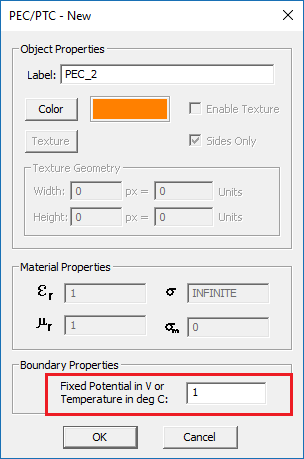The property dialog of fixed-potential PEC with a 1V voltage.

Activate each PEC group by right-clicking on their name in the navigation tree and selecting Activate from the contextual menu. Then draw two rectangle objects with the location and dimensions given in the table below.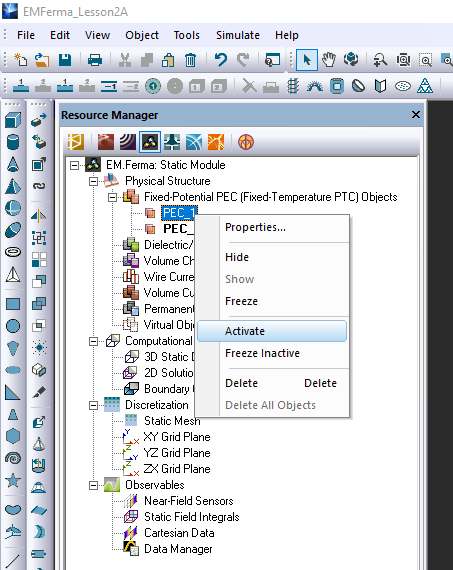Activating PEC group in the navigation tree.
Part Object Type Material Type Dimensions Coordinates Rotation Angles
Rect_Strip_1 Rectangle Strip PEC_1 50mm × 50mm (0, 0, -1mm) (0°, 0°, 0°)
Rect_Strip_2 Rectangle Strip PEC_2 50mm × 50mm (0, 0, 1mm) (0°, 0°, 0°)

To draw a rectangle, click the Rectangle Strip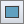button of the Object Toolbar or select the menu item Object → Surface → Rectangle Strip.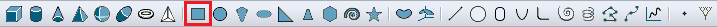Selecting the Rectangle Strip tool in the object toolbar.

With the rectangle strip tool selected, click on a blank space in the project workspace and drag the mouse to draw the planar rectangle object. A property dialog pops up at the lower right corner of your screen. As you drag the mouse, you will see that the X-dimension and Y-dimension of your new object continuously change. You can enter any values for the side dimensions or the X, Y, Z coordinates of the center of local coordinate system (LCS) at any time.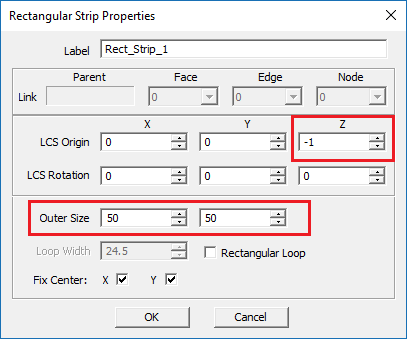The property dialog of rectangle strip.

Your physical structure should look like this: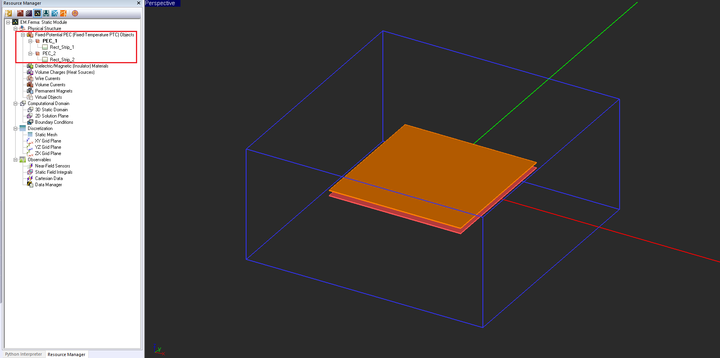The two metallic plate objects with different potentials and the default domain box enclosing them.

## Computational Domain, Boundary Conditions & Observables

You saw in the previous tutorial lesson that EM.Ferma automatically places a domain box around your objects. By default, this is a PEC box consisting of six perfect metal plates held at zero potential. In other words, the Dirichlet boundary conditions are applied to your boundary-value problem. The default domain box is placed at a certain offset distance from the largest bounding box enclosing all of your geometric objects in the project workspace. EM.Ferma's default domain offset is 20 project units. You can change this offset value individually along the six axial directions. Open the Domain Settings Dialog by clicking the 3D Static Domain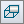button of Simulate Toolbar or by selecting the menu item Simulate → Computational Domain → Domain Settings… or using the keyboard shortcut Ctrl+A).

In this project, the bottom capacitor plate is held at zero potential, the same as the six domain boundary walls. On the other hand, the top capacitor plate has a potential of 1V. If the domain walls are placed too close to the top plate, strong field lines will establish between them. You need to place the domain walls far enough from the top plate to make them "invisible". Set the six offset values according to the table below:

Location Offset Type
-X 20mm PEC
+X 20mm PEC
-Y 20mm PEC
+Y 20mm PEC
-Z 10mm PEC
+Z 30mm PEC

Next, right-click on the Boundary Conditions item under the "Computational Domain" section of the navigation tree and open its settings dialog from the contextual menu. As you can see from the figure below, all the six walls of the domain box have a zero voltage.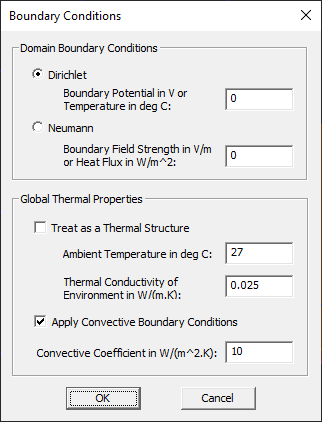EM.Ferma's boundary conditions dialog.

Finally, define two field sensor observables: the first Z-directed and called "Sensor_1", and the second X-directed and called "Sensor_2". Leave the default center coordinates of (0, 0, 0) for both sensor planes.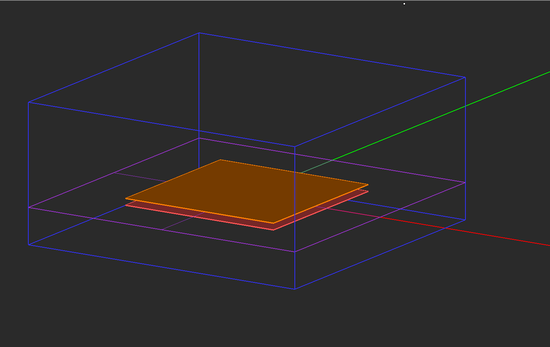The capacitor geometry with the modified domain box and two field sensor planes.

## Running an Electrostatic Analysis of Your Capacitor

At this time, your project is ready for simulation. Open the mesh settings dialog and change the Fixed Mesh Cell Size to Δx = Δy = Δz = 0.5mm, i.e. a uniform mesh along all the three principal directions. The mesh resolution was chosen so that four cells are placed between the two metal plates across the gap region.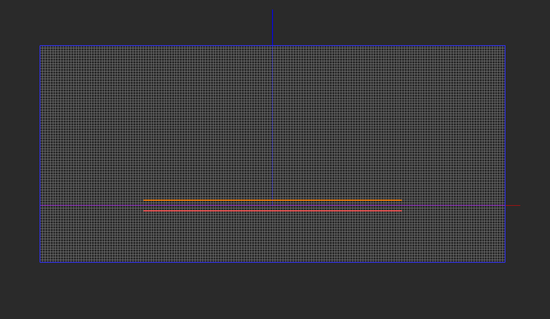The left view of the mesh of the parallel plate capacitor structure.

Run an electrostatic "Analysis" of your structure and visualize the electric field and electric potential results on both sensor planes.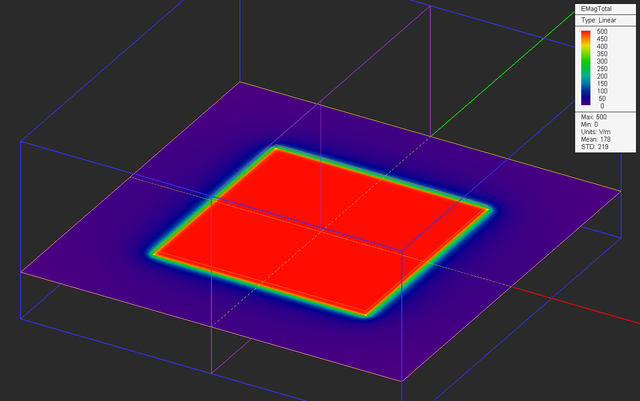The electric field distribution on the horizontal Sensor_1 (XY) plane.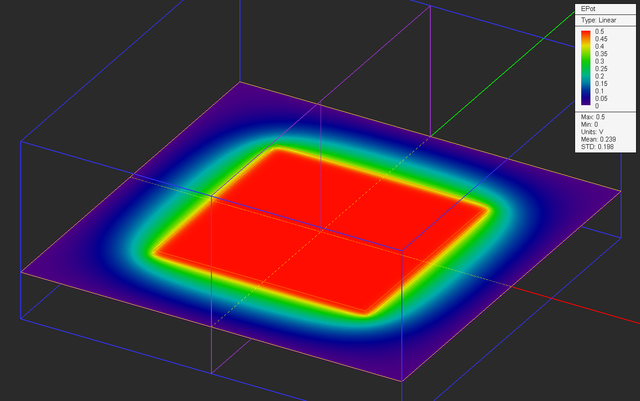The electric potential distribution on the horizontal Sensor_1 (XY) plane.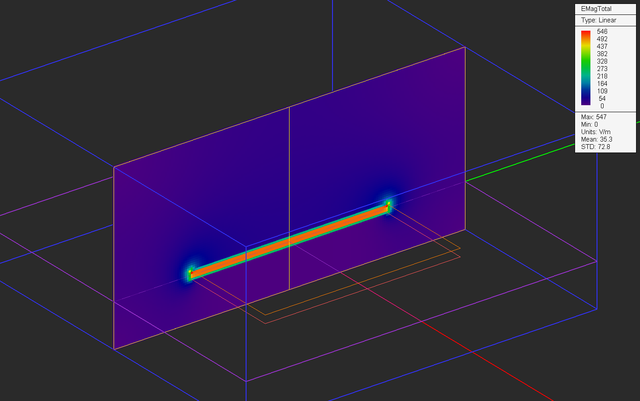The electric field distribution on the vertical Sensor_2 (YZ) plane.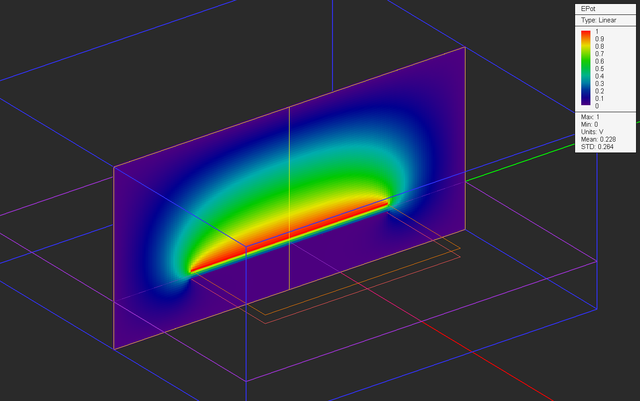The electric potential distribution on the vertical Sensor_2 (YZ) plane.

Also, open the Data Manager and plot the data file. As you should have expected, the results show a quite uniform electric field at the center of the parallel plate capacitor.

## Verifying the Simulation Results

Here we review a simple, approximate, analytical solution of the capacitor problem. Given the large size of the metal plates and their X- and Y-symmetries, one can assume that the electric potential is only a function of the z coordinate at the center. The Laplace equation can then be written as:

$\nabla^2 \Phi = \frac{d^2\Phi}{dz^2} = 0$

subject to the boundary conditions: Φ(z=-0.001m) = 0V and Φ(z = 0.001m) = 1V. The solution to this equation is:

$\Phi (z) = Az+B = 500z +0.5$

The electric field is then found to be:

$E_z = -\nabla \Phi = -\frac{d\Phi}{dz} = -500 V/m$

Open the Data Manager and plot the data files “Sensor_1_X_ETotal.DAT” and “Sensor_1_X_EPotential.DAT” as shown below. You can clearly see a wide region of constant potential Ψ = 0.5V and of constant electric field strength |E| = 500V/m under the two metal plates.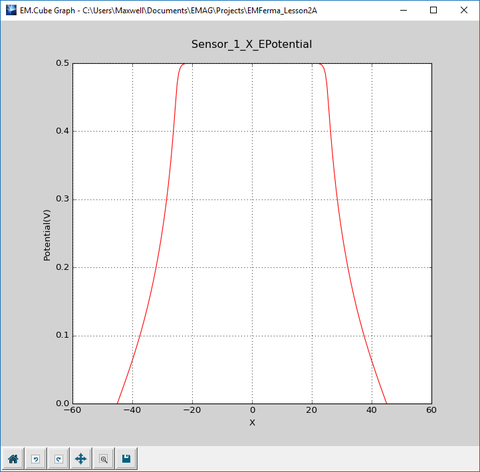Variation of the electric potential of the capacitor along the X-axis.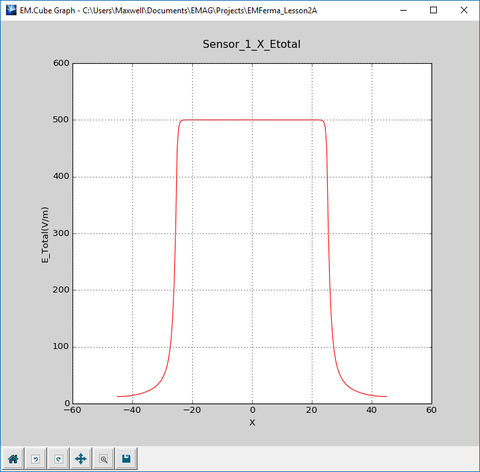Variation of the electric field of the capacitor along the X-axis.

## Defining a Field Integral Observable for Computing the Capacitance

EM.Ferma lets you define a number of post-processed observables called "Field Integrals", which are calculated by integrating field quantities over paths, loops, open or closed surfaces, etc. In this tutorial lesson, you will define a "Capacitance" observable. The capacitance is defined as:

$C = \frac{\Phi_E}{V} = \frac{\int\int_{S_o} \epsilon \mathbf{E(r)} . \mathbf{ds} } {\int_{\Gamma} \mathbf{E(r)} . \mathbf{dl} }$

where Γ represents an open curve (path), So represents a closed surface like a box, E(r) is the electric field, ΦE is the electric flux passing through So, and V is the voltage along the path Γ. In EM.Ferma, So is defined as a box characterized by two opposite corner points, and Γ is defined a line segment characterized by two endpoints.

To define the capacitance observable, right-click on the Static Field Integrals item in the "Observables" section of the navigation tree and select Insert New Field Integral… from the contextual menu. At the top of the field integral dialog, select Capacitance from the drop-down list labeled Integral Type. Also, choose turquoise as the color of the observable.

This dialog has two more sections, one for defining the domain of the electric flux surface integral, and the other for defining the path of the voltage line integral. Define the two integral domains as follows:

Integral Domain Domain Type Point 1 Coordinates Point 2 Coordinates
Electric Flux Box Closed Surface (-30mm, -30mm, 0) (-30mm, -30mm, 5mm)
Voltage Line Open Curve Path (0, 0, -1mm) (0, 0, 1mm)

For this part, delete the two field sensor observables "Sensor_1" and "Sensor_2". Right-click on the name of each one in the navigation tree and select Delete from the contextual menu. Or right-click on the topmost "Near-Field Sensors" item in the navigation tree and select Delete All from the contextual menu. Note that the flux box has been defined to enclose the upper metal plate only. The voltage path has been defined as a vertical line joining the centers of the two metal plates.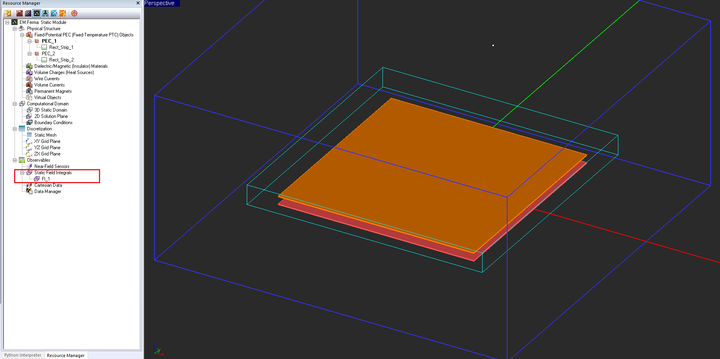The voltage line and flux box defined for the parallel plate capacitor structure.

Freeze the physical objects to see other objects hidden behind, beneath or inside it. In the figure below, the two plates have been shown in freeze state.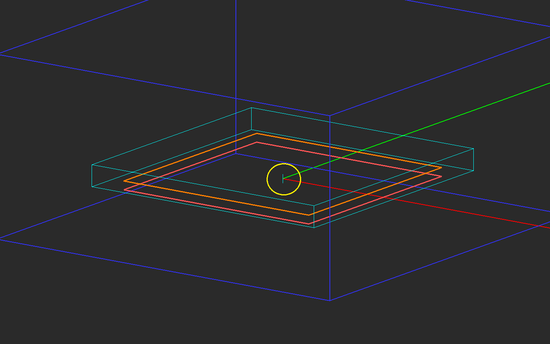The voltage line and flux box shown with the physical objects in a freeze state. The voltage line has been marked inside the yellow circle.

Before running a new simulation, open the mesh settings dialog once again and change the mesh cell size along the Z-axis to 0.2mm. This will generate a total of 10 cells across the the gap region of the capacitor and will provide a much better resolution.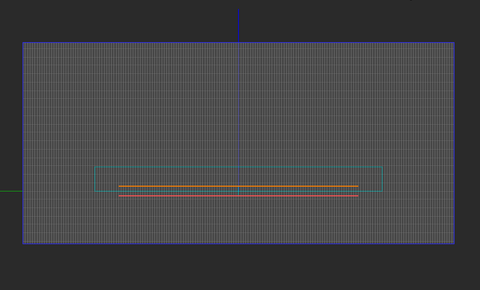Increasing the mesh resolution in the mesh settings dialog.

Run a new electrostatic analysis of the structure with the new field integral observable. At the end of the simulation, open the data manager and select the data file "FI_1_Capacitance.DAT". You can use the View button of the data manager to view the contents of this file in the data manager's built-in spreadsheet. If you do so, you will see a zero value. This is because the value of the capacitance is extremely small. While in the data manager dialog, select "FI_1_Capacitance.DAT" and click the Notepad or Open button. This opens the Windows Notepad, where you will see a value of "1.49196e-011" or 14.92pF.

Using the well-known parallel-plate capacitance formula, you will get:

$C = \epsilon_0 \frac{A}{d} = 8.8542\times 10^{-12} \left( \frac{0.05^2}{0.002} \right) = 1.1067\times 10^{-11} F = 11.06pF$

In the above formula, A is the surface area of the capacitor plates and d is the spacing between them. Note that the discrepancy is due to the assumption by the formula that the electric field is constant, uniform and strictly confined between the two parallel plates of the capacitor. However, from the field distribution plots you got earlier, it is evident that this assumption might not be very accurate.

Next, you will add a cubic dielectric filler block that will be sandwiched between the two metal plates of your capacitor structure. To do so, you need to create a dielectric material group. Right-click on the Dielectric/Magnetic Materials item in the navigation tree and select Insert New Dielectric... from the contextual menu. This opens up the New Dielectric dialog. You can choose a name for your material and assign material properties like permittivity.

Or you can use EM.Ferma's material database. Click the Material button to open the Materials List. For this project, choose "Mica" with a permittivity of εr = 5.4. In the Materials Dialog, you can simply type in the letter "M" and it will take you to the materials whose name starts with that letter.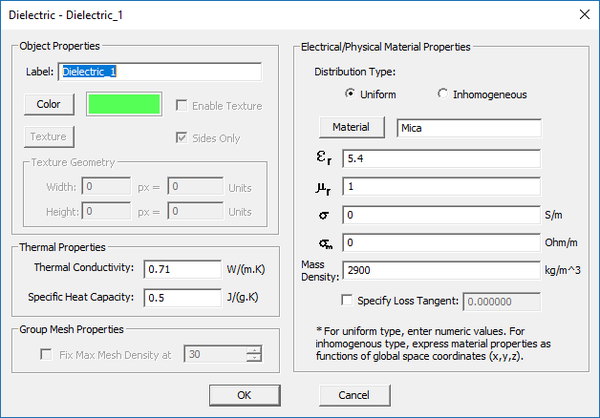The property dialog of the dielectric material group with Mica selected.

To draw a box object, click the Box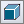button of the Object Toolbar or select the menu item Object → Solid → Box.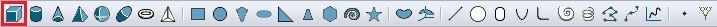Selecting the Box Tool from the Object Toolbar.

With the Box tool selected, click on a blank space in the project workspace and drag the mouse to draw the rectangular base of your box object. A property dialog pops up at the lower right corner of your screen. As you drag the mouse, you will see that the X-dimension and Y-dimension of your new object continuously change. When the base reaches the desired size or something close to that, click the mouse. Next, you have to give the right height to your box. Drag the mouse upward until you reach the desired height (Z-dimension). Then, click once more (i.e. drop the mouse). At this time, the drawing of your box is complete. You can always enter values for the dimensions or LCS coordinates at any time. While the new Dielectric_1 group is active, draw a box object according to the table below:

Part Object Type Material Type Dimensions Coordinates Rotation Angles
Box_1 Box Dielectric_1 50mm × 50mm × 2mm (0, 0, -1mm) (0°, 0°, 0°)

Your dielectric-filled parallel plate capacitor should now look like this: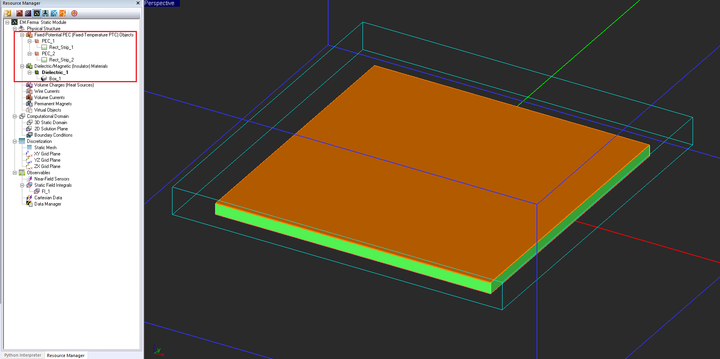The geometry of the dielectric-filled parallel plate capacitor with the flux box.

With the same domain box, mesh settings and observables from the previous part, run a new electrostatic analysis of your structure. The electric field and potential distributions will be very similar to the case of air-filled capacitor. That is why you didn't initiate new field sensor observables for this part.

Open the data manager and view the contents of the data file "FI_1_Capacitance.DAT" in Windows Notepad. This time you will see that the value of the capacitance has increased to "6.79847e-011" or 67.98pF. The parallel-plate capacitance formula with a dielectric spacer would yield:

$C = \epsilon_r \epsilon_0 \frac{A}{d} = 5.4 \times 8.854\times 10^{-12} \left( \frac{0.05^2}{0.002} \right) = 5.976\times 10^{-11} F = 59.76pF$

The better agreement in this case (12% deviation) is clearly due to the fact that the dielectric filler helps increased confinement of the electric field in the space between the parallel plates.